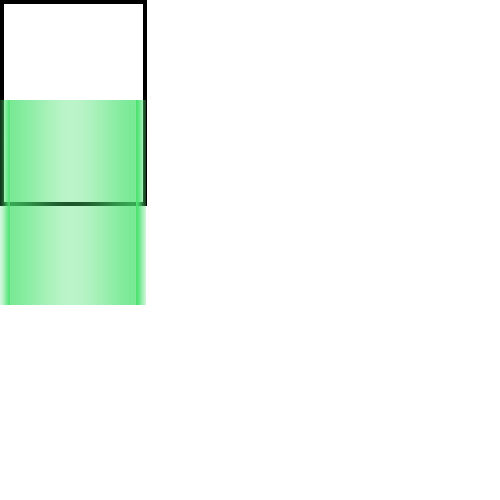# （Pillow）Affine 图形变换的矩阵参数详解

## 二、渲染方法¶

img2 = img.transform(bg.size, Image.AFFINE, (1, -0.5, 0, 0, 2, 0))


• bg.size 是最终图像的裁剪大小，在变换完成后，Pillow 会将图片裁剪至该大小。
• Image.AFFINE 是变换类型，这里是仿射变换。
• (1, -0.5, 0, 0, 2, 0) 是变换矩阵，用于描述仿射变换的参数。

## 三、变换矩阵¶

$\begin{bmatrix} xScale & xSkew & xTranslate \\ ySkew & yScale & yTranslate \\ 0 & 0 & 1 \\ \end{bmatrix}$

matrix = (xScale, xSkew, xTranslate, ySkew, yScale, yTranslate)


## 四、矩阵各值对 Pillow 实际图像的影响¶

from PIL import Image, ImageDraw

bg = Image.new('RGBA', (500, 500), (255, 255, 255, 0))
img = Image.open('longNoteLine2.png').convert('RGBA')

# 绘制原图轮廓，以便观察变换
outline = Image.new('RGBA', bg.size, (255, 255, 255, 0))
draw = ImageDraw.Draw(outline)
draw.rectangle((0, 0, 0 + img.size, 0 + img.size), fill=(0, 0, 0, 0), width=4, outline=(0, 0, 0, 255))
bg.alpha_composite(outline, (0, 0))

# 进行变换
img2 = img.transform(bg.size, Image.AFFINE, (1, 0, 0, 0, 1, 0))

bg.alpha_composite(img2, (0, 0))
bg.show()


### 4.1 x 轴缩放系数 xScale¶

(1, 0, 0, 0, 1, 0)(2, 0, 0, 0, 1, 0)

xScale == 2，将图像在 x 轴方向上缩小至原来的 1/2。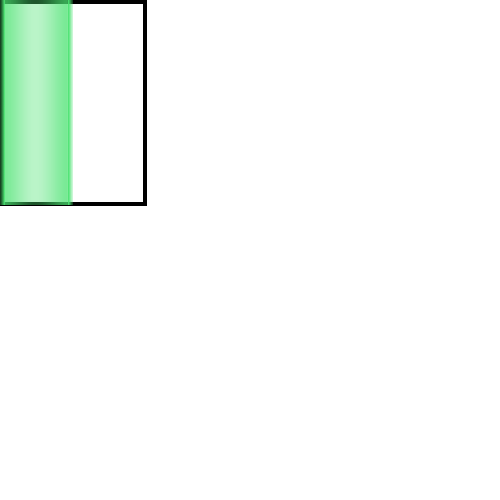(0.5, 0, 0, 0, 1, 0)

xScale == 0.5，将图像在 x 轴方向上放大至原来的 2 倍。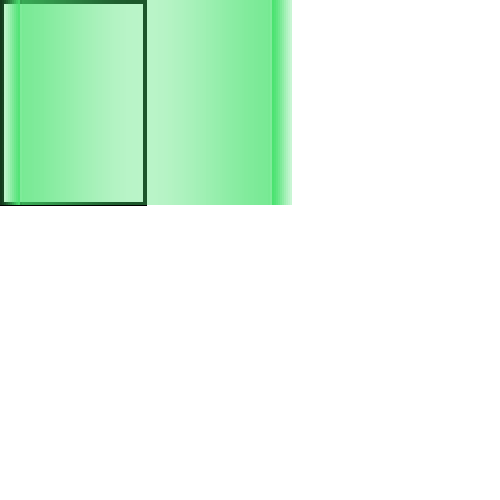### 4.2 x 轴旋转系数 xSkew¶

(1, 1, 0, 0, 1, 0)

xSkew == tan(45°) == 1，保持上底边位置不变，将平行四边形下底边在 x 轴方向倾斜至 45°。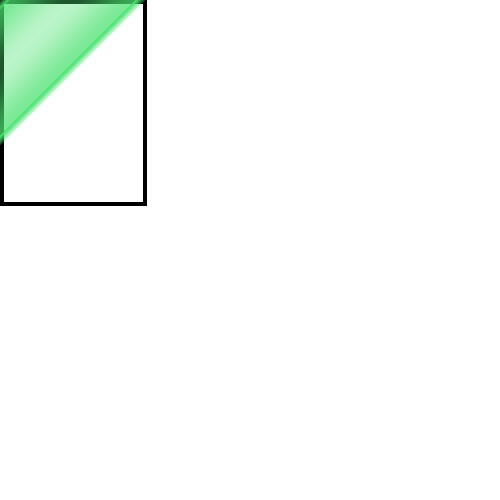(1, -1, 0, 0, 1, 0)

xSkew == tan(-45°) == -1，保持上底边位置不变，将平行四边形下底边在 x 轴方向倾斜至 45°。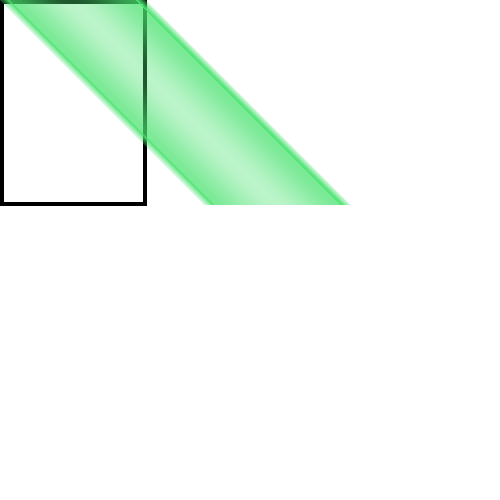### 4.3 x 轴平移系数 xTranslate¶

(1, 0, 100, 0, 1, 0)

xTranslate == 100，将图像在 x 轴方向上平移 100 像素。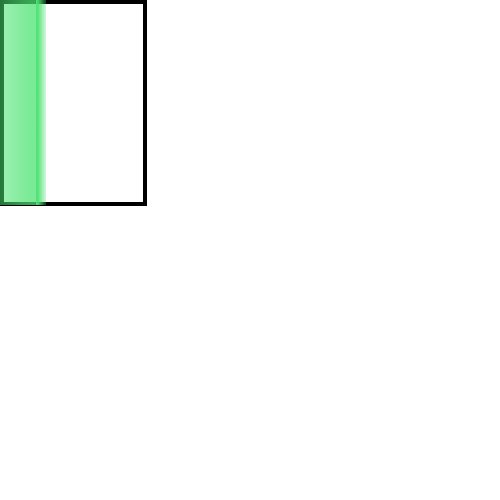(1, 0, -100, 0, 1, 0)

xTranslate == -100，将图像在 x 轴方向上平移 100 像素。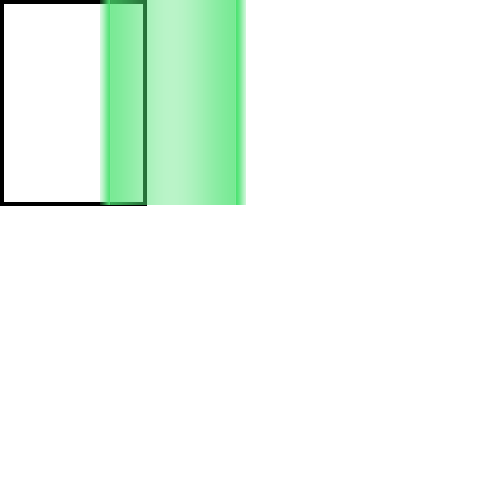### 4.4 y 轴旋转系数 ySkew¶

(1, 0, 0, 1, 1, 0)

ySkew == tan(45°) == 1，保持左底边位置不变，将平行四边形右底边在 y 轴方向斜切至 45°。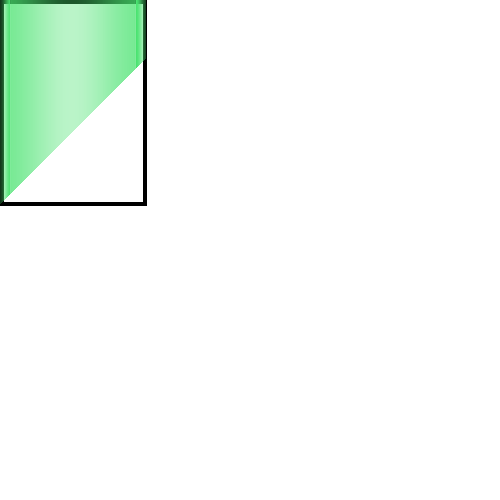(1, 0, 0, -1, 1, 0)

ySkew == tan(-45°) == -1，保持左底边位置不变，将平行四边形右底边在 y 轴方向斜切至 45°。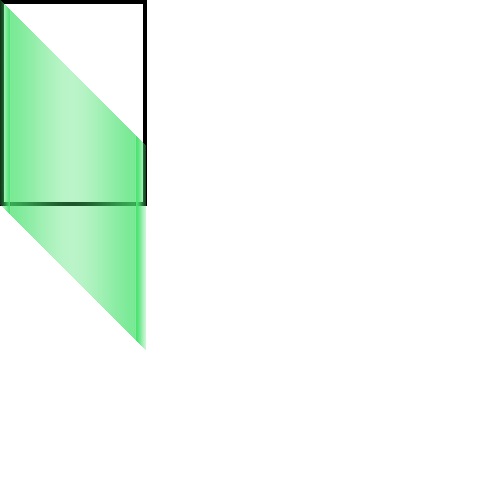### 4.5 y 轴缩放系数 yScale¶

(1, 0, 0, 0, 2, 0)

yScale == 2，将图像在 y 轴方向上缩小至原来的 1/2。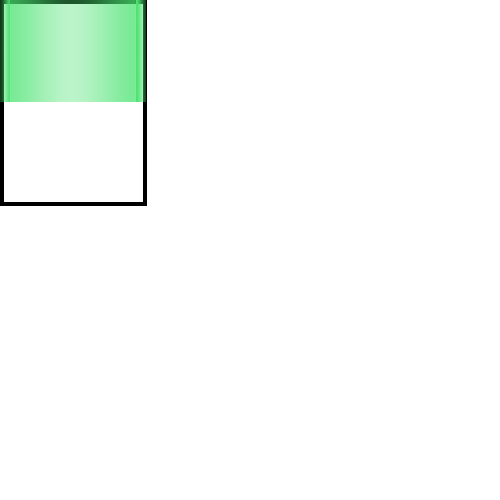(1, 0, 0, 0, 0.5, 0)

yScale == 0.5，将图像在 y 轴方向上放大至原来的 2 倍。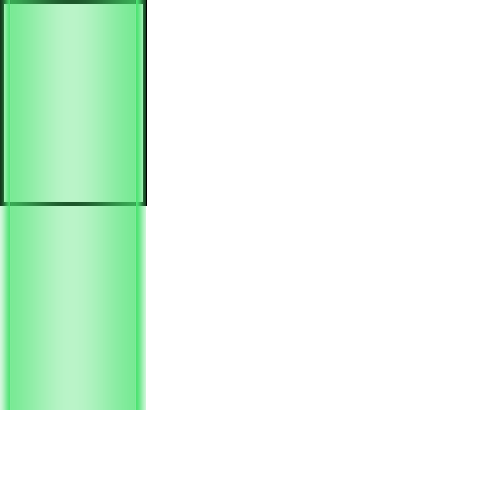### 4.6 y 轴平移系数 yTranslate¶

(1, 0, 0, 0, 1, 100)

yTranslate == 100，将图像在 y 轴方向上平移 100 像素。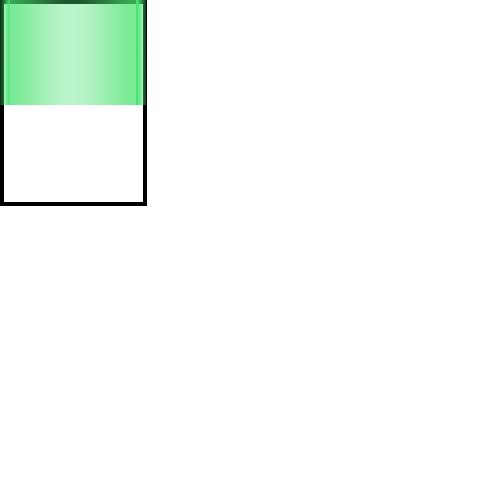(1, 0, 0, 0, 1, -100)

yTranslate == -100，将图像在 y 轴方向上平移 100 像素。